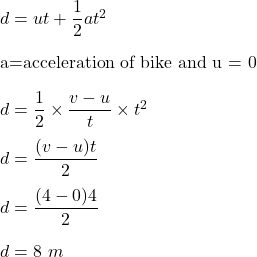A bike accelerates from 0.0 m/s to 4.0 m/s in 4 s. What distance does it travel?

Question

A bike accelerates from 0.0 m/s to 4.0 m/s in

4 s. What distance does it travel?

in progress 0
6 months 2021-07-14T04:00:28+00:00 1 Answers 51 views 0

d = 8 m

Explanation:

Given that,

Initial speed, u = 0

Final speed, v = 4 m/s

Time, t = 4 s

We need to find the distance traveled by a bike. Let it is equal to d. Using second equation of kinematics as follows :So, the car cover a distance of 8 m.